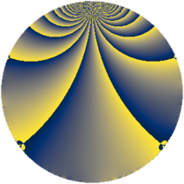# Properties

 Label 441.2.sLevel $441$ Weight $2$ Character orbit 441.s Rep. character $\chi_{441}(362,\cdot)$ Character field $\Q(\zeta_{6})$ Dimension $72$ Newform subspaces $4$ Sturm bound $112$ Trace bound $1$

# Learn more

## Defining parameters

 Level: $$N$$ $$=$$ $$441 = 3^{2} \cdot 7^{2}$$ Weight: $$k$$ $$=$$ $$2$$ Character orbit: $$[\chi]$$ $$=$$ 441.s (of order $$6$$ and degree $$2$$) Character conductor: $$\operatorname{cond}(\chi)$$ $$=$$ $$63$$ Character field: $$\Q(\zeta_{6})$$ Newform subspaces: $$4$$ Sturm bound: $$112$$ Trace bound: $$1$$ Distinguishing $$T_p$$: $$2$$

## Dimensions

The following table gives the dimensions of various subspaces of $$M_{2}(441, [\chi])$$.

Total New Old
Modular forms 128 88 40
Cusp forms 96 72 24
Eisenstein series 32 16 16

## Trace form

 $$72q + 3q^{2} + 3q^{3} + 31q^{4} + 6q^{5} + 6q^{6} - 5q^{9} + O(q^{10})$$ $$72q + 3q^{2} + 3q^{3} + 31q^{4} + 6q^{5} + 6q^{6} - 5q^{9} + 6q^{10} + 3q^{12} - 3q^{13} - 28q^{15} - 23q^{16} + 9q^{17} + 23q^{18} + 6q^{19} + 6q^{20} - 8q^{22} - 24q^{24} + 42q^{25} - 6q^{26} - 27q^{27} + 6q^{29} + 12q^{30} + 15q^{31} - 69q^{32} + 6q^{33} + 6q^{34} - 2q^{36} + q^{37} - 54q^{38} - 26q^{39} + 6q^{41} - 8q^{43} + 69q^{44} - 6q^{45} + 16q^{46} - 15q^{47} + 15q^{48} + 3q^{50} + 42q^{51} + 36q^{53} - 36q^{54} + 35q^{57} - 2q^{58} + 18q^{59} - 96q^{60} - 36q^{61} - 24q^{62} - 28q^{64} - 36q^{65} + 33q^{66} + 6q^{67} + 48q^{68} + 12q^{69} + 5q^{72} + 6q^{73} + 33q^{75} - 85q^{78} - 18q^{79} + 45q^{80} + 87q^{81} + 30q^{83} - 21q^{85} - 39q^{87} - 46q^{88} - 27q^{89} - 51q^{90} - 84q^{92} - 26q^{93} + 3q^{94} - 141q^{95} - 12q^{96} - 3q^{97} - 84q^{99} + O(q^{100})$$

## Decomposition of $$S_{2}^{\mathrm{new}}(441, [\chi])$$ into newform subspaces

Label Dim. $$A$$ Field CM Traces $q$-expansion
$$a_2$$ $$a_3$$ $$a_5$$ $$a_7$$
441.2.s.a $$2$$ $$3.521$$ $$\Q(\sqrt{-3})$$ None $$-3$$ $$3$$ $$6$$ $$0$$ $$q+(-1-\zeta_{6})q^{2}+(2-\zeta_{6})q^{3}+\zeta_{6}q^{4}+\cdots$$
441.2.s.b $$10$$ $$3.521$$ 10.0.$$\cdots$$.1 None $$0$$ $$0$$ $$0$$ $$0$$ $$q+(\beta _{4}+\beta _{7}+\beta _{8})q^{2}+(\beta _{1}-\beta _{2}+\beta _{4}+\cdots)q^{3}+\cdots$$
441.2.s.c $$12$$ $$3.521$$ $$\mathbb{Q}[x]/(x^{12} - \cdots)$$ None $$6$$ $$0$$ $$0$$ $$0$$ $$q+\beta _{1}q^{2}-\beta _{10}q^{3}+(\beta _{1}+\beta _{4}+\beta _{7}+\cdots)q^{4}+\cdots$$
441.2.s.d $$48$$ $$3.521$$ None $$0$$ $$0$$ $$0$$ $$0$$

## Decomposition of $$S_{2}^{\mathrm{old}}(441, [\chi])$$ into lower level spaces

$$S_{2}^{\mathrm{old}}(441, [\chi]) \cong$$ $$S_{2}^{\mathrm{new}}(63, [\chi])$$$$^{\oplus 2}$$